# RS Aggarwal Solutions for Class 6 Chapter 9 Linear Equations in One Variable Exercise 9B

The fundamental concepts which are explained in Class 6 are important as they are continued in higher grades also. RS Aggarwal Solutions which are prepared by faculty at BYJU’S are relevant to the CBSE guidelines of Class 6. Exercise 9B contains problems which help in solving equations using a systematic method. The students can practice the exercise wise problems using the PDF of solutions to ace the exam. Students can download RS Aggarwal Solutions for Class 6 Chapter 9 Linear Equations in One Variable Exercise 9B PDF here.

## Download PDF of RS Aggarwal Solutions for Class 6 Chapter 9 Linear Equations in One Variable Exercise 9B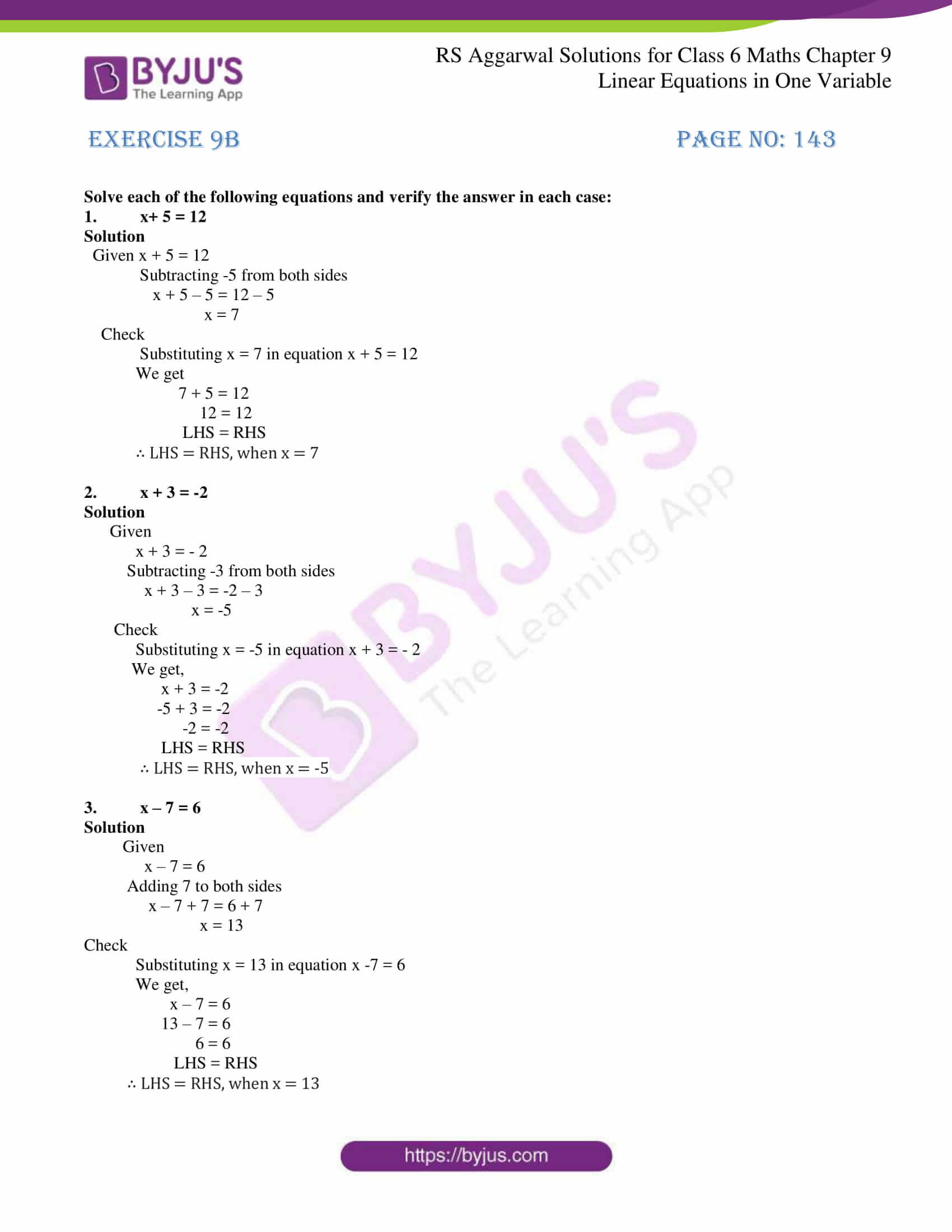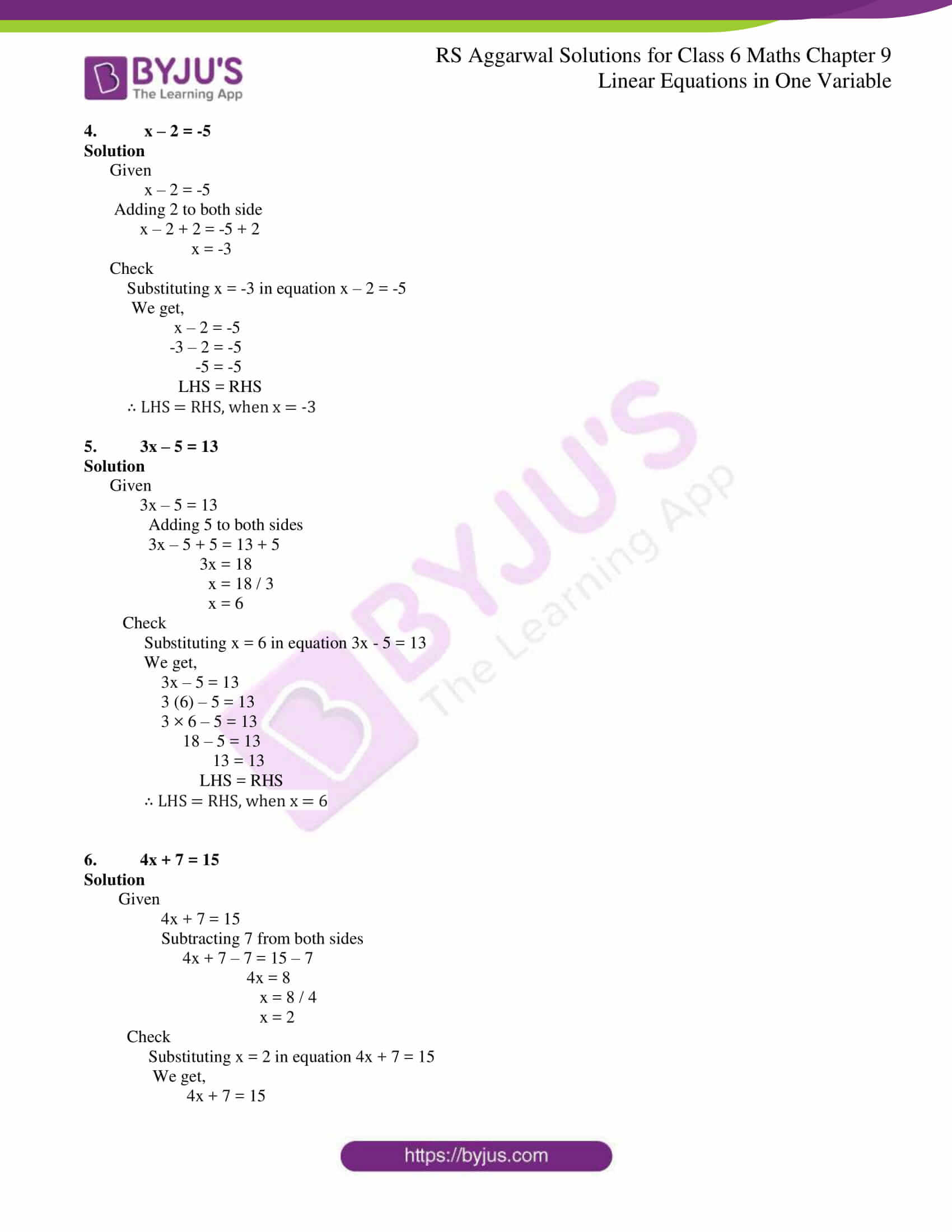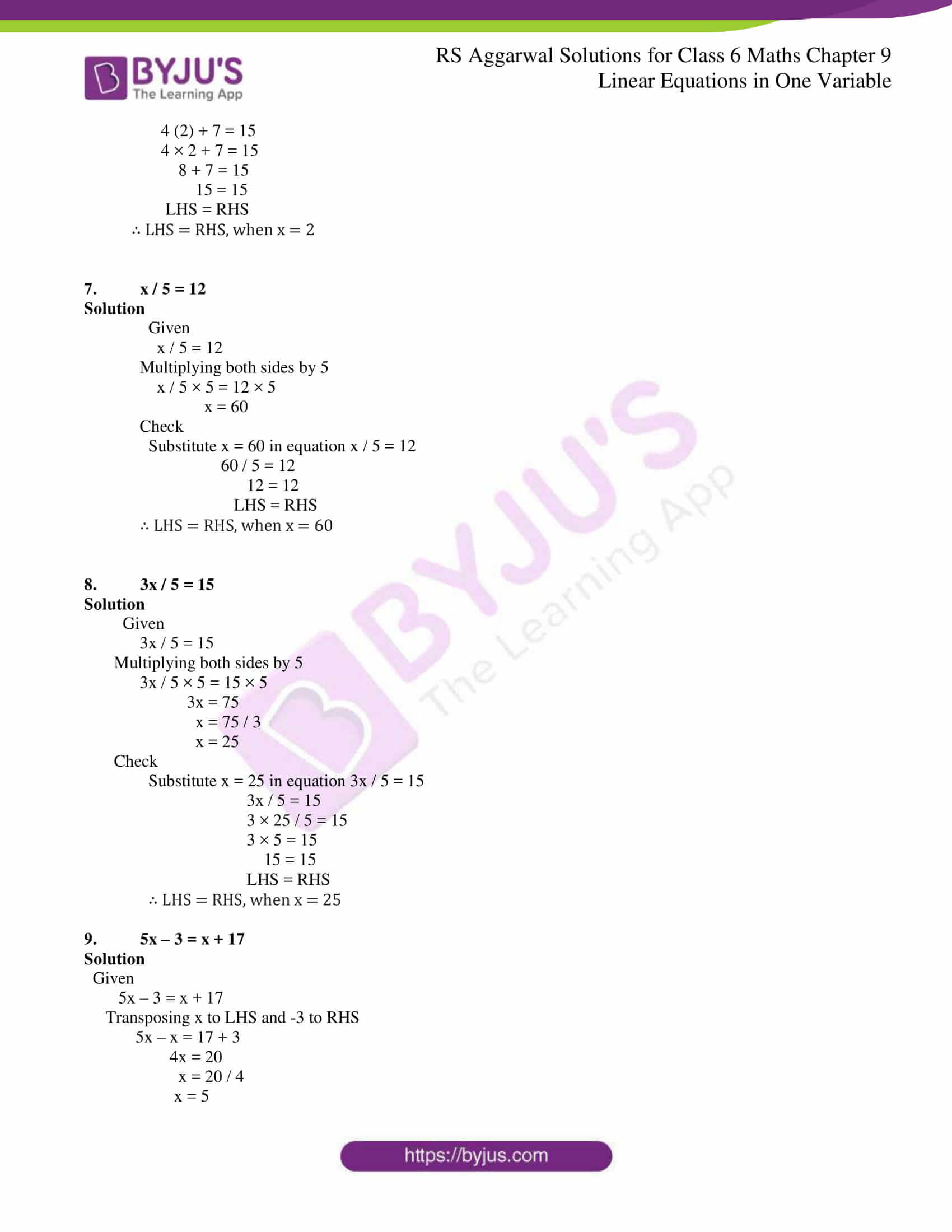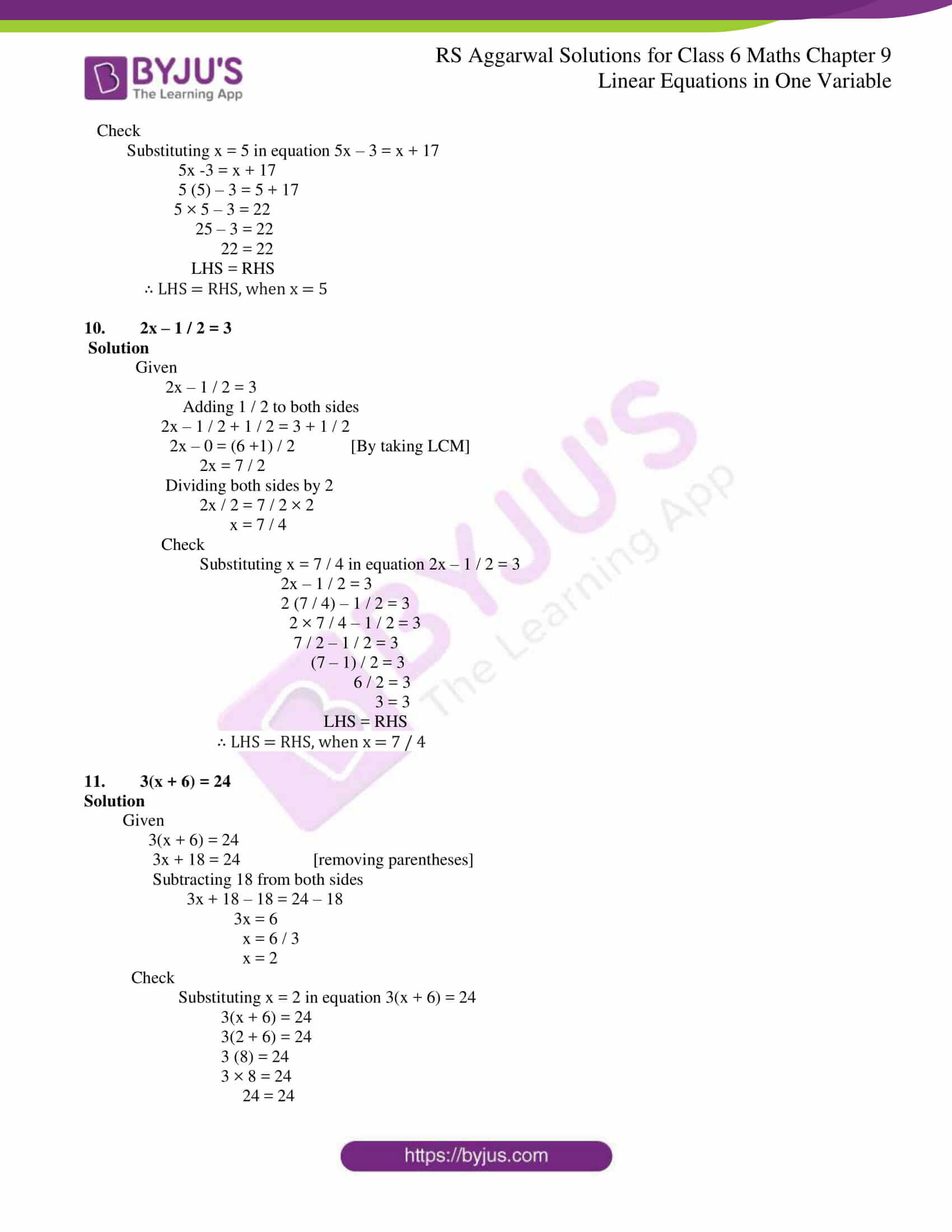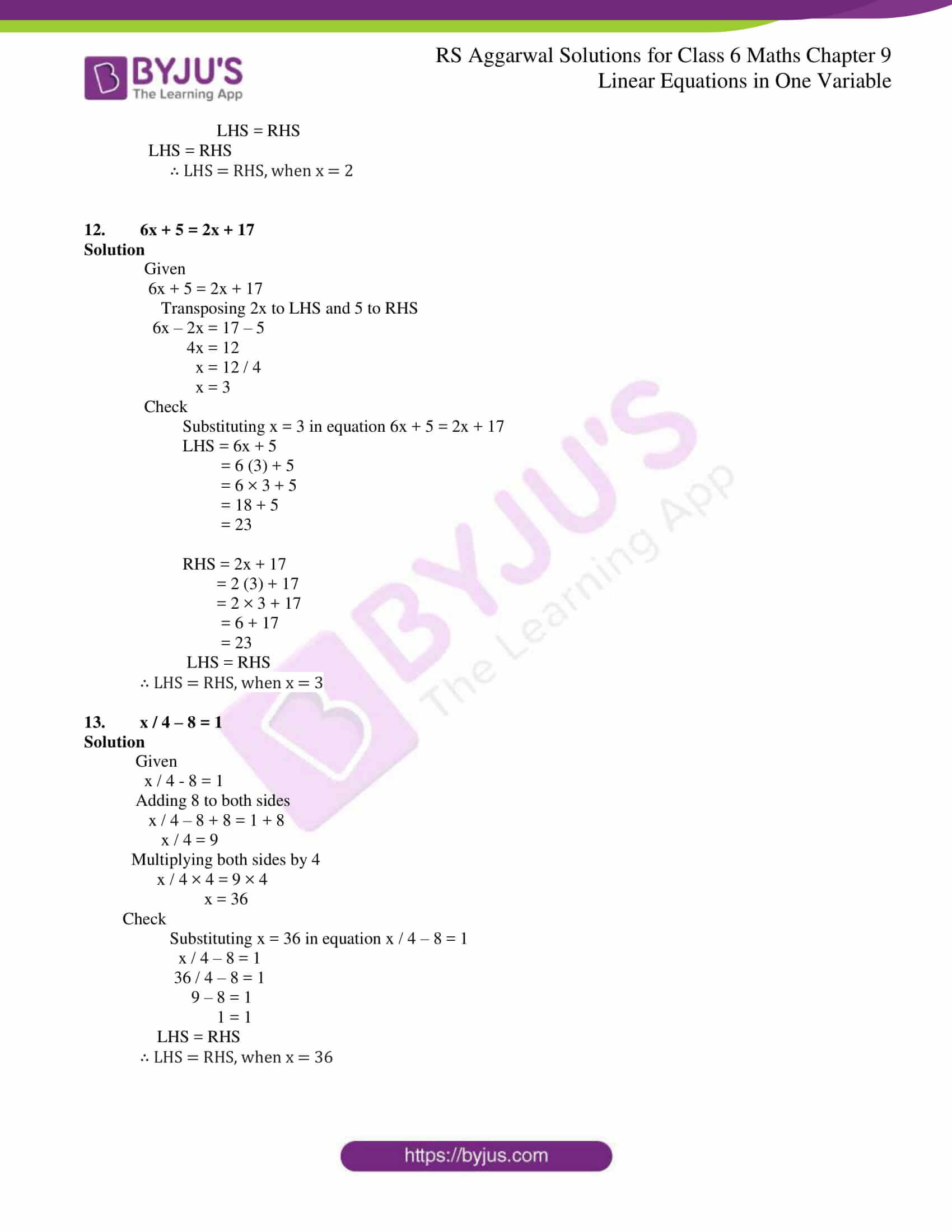### Access answers to Maths RS Aggarwal Solutions for Class 6 Chapter 9 Linear Equations in One Variable Exercise 9B

Solve each of the following equations and verify the answer in each case:

1. x+ 5 = 12

Solution

Given x + 5 = 12

Subtracting -5 from both sides

x + 5 – 5 = 12 – 5

x = 7

Check

Substituting x = 7 in equation x + 5 = 12

We get

7 + 5 = 12

12 = 12

LHS = RHS

∴ LHS = RHS, when x = 7

2. x + 3 = -2

Solution

Given

x + 3 = – 2

Subtracting -3 from both sides

x + 3 – 3 = -2 – 3

x = -5

Check

Substituting x = -5 in equation x + 3 = – 2

We get,

x + 3 = -2

-5 + 3 = -2

-2 = -2

LHS = RHS

∴ LHS = RHS, when x = -5

3. x – 7 = 6

Solution

Given

x – 7 = 6

x – 7 + 7 = 6 + 7

x = 13

Check

Substituting x = 13 in equation x -7 = 6

We get,

x – 7 = 6

13 – 7 = 6

6 = 6

LHS = RHS

∴ LHS = RHS, when x = 13

4. x – 2 = -5

Solution

Given

x – 2 = -5

x – 2 + 2 = -5 + 2

x = -3

Check

Substituting x = -3 in equation x – 2 = -5

We get,

x – 2 = -5

-3 – 2 = -5

-5 = -5

LHS = RHS

∴ LHS = RHS, when x = -3

5. 3x – 5 = 13

Solution

Given

3x – 5 = 13

3x – 5 + 5 = 13 + 5

3x = 18

x = 18 / 3

x = 6

Check

Substituting x = 6 in equation 3x – 5 = 13

We get,

3x – 5 = 13

3 (6) – 5 = 13

3 × 6 – 5 = 13

18 – 5 = 13

13 = 13

LHS = RHS

∴ LHS = RHS, when x = 6

6. 4x + 7 = 15

Solution

Given

4x + 7 = 15

Subtracting 7 from both sides

4x + 7 – 7 = 15 – 7

4x = 8

x = 8 / 4

x = 2

Check

Substituting x = 2 in equation 4x + 7 = 15

We get,

4x + 7 = 15

4 (2) + 7 = 15

4 × 2 + 7 = 15

8 + 7 = 15

15 = 15

LHS = RHS

∴ LHS = RHS, when x = 2

7. x / 5 = 12

Solution

Given

x / 5 = 12

Multiplying both sides by 5

x / 5 × 5 = 12 × 5

x = 60

Check

Substitute x = 60 in equation x / 5 = 12

60 / 5 = 12

12 = 12

LHS = RHS

∴ LHS = RHS, when x = 60

8. 3x / 5 = 15

Solution

Given

3x / 5 = 15

Multiplying both sides by 5

3x / 5 × 5 = 15 × 5

3x = 75

x = 75 / 3

x = 25

Check

Substitute x = 25 in equation 3x / 5 = 15

3x / 5 = 15

3 × 25 / 5 = 15

3 × 5 = 15

15 = 15

LHS = RHS

∴ LHS = RHS, when x = 25

9. 5x – 3 = x + 17

Solution

Given

5x – 3 = x + 17

Transposing x to LHS and -3 to RHS

5x – x = 17 + 3

4x = 20

x = 20 / 4

x = 5

Check

Substituting x = 5 in equation 5x – 3 = x + 17

5x -3 = x + 17

5 (5) – 3 = 5 + 17

5 × 5 – 3 = 22

25 – 3 = 22

22 = 22

LHS = RHS

∴ LHS = RHS, when x = 5

10. 2x – 1 / 2 = 3

Solution

Given

2x – 1 / 2 = 3

Adding 1 / 2 to both sides

2x – 1 / 2 + 1 / 2 = 3 + 1 / 2

2x – 0 = (6 +1) / 2 [By taking LCM]

2x = 7 / 2

Dividing both sides by 2

2x / 2 = 7 / 2 × 2

x = 7 / 4

Check

Substituting x = 7 / 4 in equation 2x – 1 / 2 = 3

2x – 1 / 2 = 3

2 (7 / 4) – 1 / 2 = 3

2 × 7 / 4 – 1 / 2 = 3

7 / 2 – 1 / 2 = 3

(7 – 1) / 2 = 3

6 / 2 = 3

3 = 3

LHS = RHS

∴ LHS = RHS, when x = 7 / 4

11. 3(x + 6) = 24

Solution

Given

3(x + 6) = 24

3x + 18 = 24 [removing parentheses]

Subtracting 18 from both sides

3x + 18 – 18 = 24 – 18

3x = 6

x = 6 / 3

x = 2

Check

Substituting x = 2 in equation 3(x + 6) = 24

3(x + 6) = 24

3(2 + 6) = 24

3 (8) = 24

3 × 8 = 24

24 = 24

LHS = RHS

LHS = RHS

∴ LHS = RHS, when x = 2

12. 6x + 5 = 2x + 17

Solution

Given

6x + 5 = 2x + 17

Transposing 2x to LHS and 5 to RHS

6x – 2x = 17 – 5

4x = 12

x = 12 / 4

x = 3

Check

Substituting x = 3 in equation 6x + 5 = 2x + 17

LHS = 6x + 5

= 6 (3) + 5

= 6 × 3 + 5

= 18 + 5

= 23

RHS = 2x + 17

= 2 (3) + 17

= 2 × 3 + 17

= 6 + 17

= 23

LHS = RHS

∴ LHS = RHS, when x = 3

13. x / 4 – 8 = 1

Solution

Given

x / 4 – 8 = 1

x / 4 – 8 + 8 = 1 + 8

x / 4 = 9

Multiplying both sides by 4

x / 4 × 4 = 9 × 4

x = 36

Check

Substituting x = 36 in equation x / 4 – 8 = 1

x / 4 – 8 = 1

36 / 4 – 8 = 1

9 – 8 = 1

1 = 1

LHS = RHS

∴ LHS = RHS, when x = 36